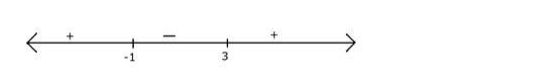# Find the solution set of the in equation

Question:

Find the solution set of the in equation $|x-1|<2$.

Solution:

$|x-1|<2$

Square both sides

$\Rightarrow(x-1)^{2}<4$

$\Rightarrow x^{2}-2 x+1<4$

$\Rightarrow x^{2}-2 x-3<0$

$\Rightarrow x^{2}-3 x+x-3<0$

$\Rightarrow x(x-3)+1(x-3)<0$

$\Rightarrow(x+1)(x-3)<0$

Observe that when $x>3(x-3)(x+1)$ is positive

And for each root the sign changes henceWe want less than 0 that is negative part

Hence $x$ should be between $-1$ and 3 for $(x-3)(x+1)$ to be negative

Hence $x \in(-1,3)$

Hence solution set for $|x-1|<2$ is $(-1,3)$